# Test: Electrical Machines- 2

## 20 Questions MCQ Test Mock Test Series for SSC JE Electrical Engineering | Test: Electrical Machines- 2

Description
Attempt Test: Electrical Machines- 2 | 20 questions in 12 minutes | Mock test for SSC preparation | Free important questions MCQ to study Mock Test Series for SSC JE Electrical Engineering for SSC Exam | Download free PDF with solutions
QUESTION: 1

### In a loaded dc motor, if the brushes are given a shift from the inter-pole axis in the direction of rotation, then the commutation will

Solution:

In a loaded dc motor, if the brushes are given a shift from the inter-pole axis in the direction of rotation, then the commutation will deteriorate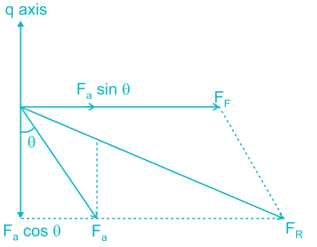The armature reaction is partially magnetizing due to horizontal component and partially cross magnetizing due to vertical component. The flux will increase and hence there will be reduction in speed

QUESTION: 2

### If two 8-pole dc machines of identical armature are wound, one with lap winding and the other with wave winding, then

Solution:

For wave wound, number of parallel paths (A) = 2

For lap wound, number of parallel paths (A) = P = 8

Hence the current rating is less in wave wound

We know that,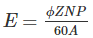Hence the voltage rating is more in wave wound

QUESTION: 3

### Consider the following statements regarding synchronous machines: 1. In an alternator, armature mmf leads the field flux 2. In an alternator, airgap flux lags the field flux 3. In a synchronous motor, armature mmf leads the field flux 4. In an alternator, field flux lags the armature current From these, the correct answer is

Solution:

1. In an alternator, armature mmf lags the field flux

2. In an alternator, airgap flux lags the field flux

3. In a synchronous motor, armature mmf leads the field flux

4. In an alternator, field flux leads the armature current

QUESTION: 4

The pitch factor, in rotating electrical machinery, is defined as the ratio of resultant emf of a

Solution:

The pitch factor is defined as the ratio of resultant emf of a short pitched coil to resultant emf of a full pitched coil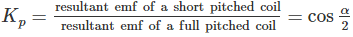QUESTION: 5

Consider the following statements:

The use of inter-poles in dc machines is to counteract the

1. Reactance voltage

2. Demagnetizing effect of armature mmf in the commutating zone

3. Cross-magnetizing effect of armature mmf in the commutating zone

Which of these statement(s) is/are correct?

Solution:

The inter-poles of appropriate polarity are strengthened so that in inter-pole zone, the cross- magnetizing effect of armature mmf is neutralized and in addition some flux is produced there. This additional flux in the inter-pole zone induces rotational emf in the commutated coil in such a direction as to oppose the reactance voltage.

QUESTION: 6

Consider the following statements: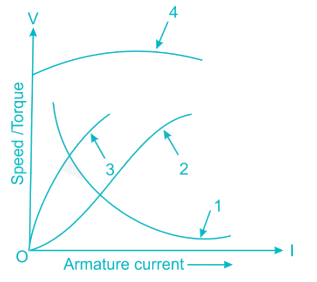In the above figure, the curves pertain to the DC motor

A. Speed vs Armature current characteristic of a DC shunt motor

B. Torque vs Armature current characteristic of a DC shunt motor

C. Speed vs Armature current characteristic of a DC series motor

D. Torque vs Armature current characteristic of a DC series motor

Solution:

The curve 1 represents Speed vs Armature current characteristic of a DC series motor

The curve 2 represents Torque vs Armature current characteristic of a DC series motor

The curve 3 represents Torque vs Armature current characteristic of a DC shunt motor

The curve 4 represents Speed vs Armature current characteristic of a DC shunt motor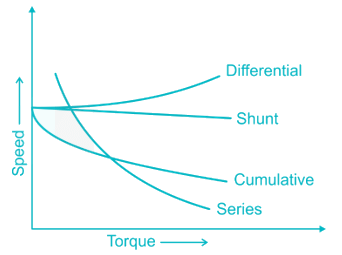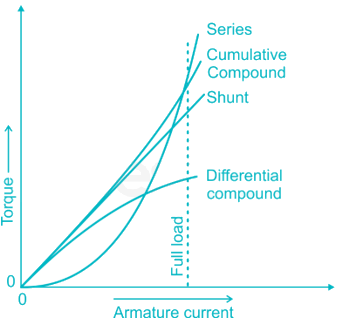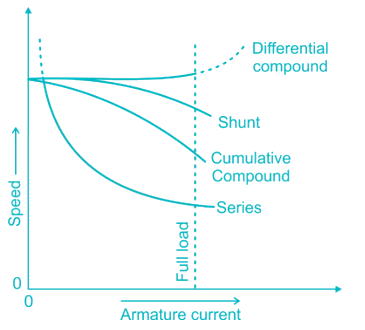QUESTION: 7

Due to low supply voltage for a 40 HP, 3-phase induction motor, which of the following equipment can be used to boost the voltage?

Solution:

A synchronous condenser is an overexcited synchronous motor, which draws leading currents from the system and hence compensates for lagging vars. It is used as a reactive power compensator in some systems for power factor correction purposes.

A tap changer is a mechanism in transformers which allows for variable turn ratios to be selected in discrete steps. These are often placed on the high voltage (low current) transformer winding for easy access and to minimize the current load during operation

Series capacitors are used to compensate the inductance of transmission line. Series capacitors will increase the transmission capacity and the stability of the line.

Shunt capacitor banks are primarily used to improve the power factor in the network. They also improve the voltage stability and reduce network losses.

Hence shunt capacitor should be used to boost the voltage.

QUESTION: 8

The injected emf in the rotor of induction motor must have

Solution:

When the speed control of three phase induction motor is done by adding resistance in rotor circuit, some part of power called, the slip power is lost. Therefore the efficiency of three phase induction motor is reduced by this method of speed control.

This slip power loss can be recovered and supplied back to improve the overall efficiency of the three-phase induction motor. This scheme of recovering the power is called slip power recovery scheme and this is done by connecting an external source of emf of slip frequency to the rotor circuit.

The injected emf can either oppose the rotor induced emf or aids the rotor induced emf. If it opposes the rotor induced emf, the total rotor resistance increases and hence the speed is decreased and if the injected emf aids the main rotor emf the total decreases and hence speed increases.

QUESTION: 9

In a split phase motor, the ratio of number of turns for starting winding to that for running winding is

Solution:

A split-phase motor has a starting winding separate from the main winding. When the motor is starting, the starting winding is connected to the power source through a centrifugal switch which is closed at low speed. The starting winding is wound with fewer turns of smaller wire than the main winding, so it has a lower inductance (L) and higher resistance (R). The lower L/R ratio creates a small phase shift, not more than about 30 degrees between the flux due to the main winding and the flux of the starting winding.

Hence the ratio of number of turns for starting winding to that for running winding is less than 1.

QUESTION: 10

A synchronous motor connected to infinite bus-bars has constant full-load, 100% excitation and unity p.f. On changing the excitation alone, the excitation current will have

Solution:

From the V curves and inverted V curves, we can say that the excitation current will have leading power factor with over excitation and lagging power factor with under excitation.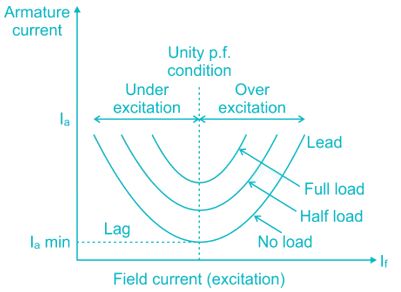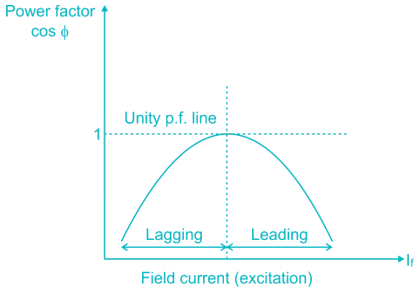QUESTION: 11

Which of the following is an AC motor?

Solution:

All the given motors (slip ring induction motor, synchronous motor, squirrel cage induction motor) are AC motors.

QUESTION: 12

If a 3-phase alternator is short-circuited at its terminal, then the initial value of short-circuit current will be limited by ________.

Solution:

If a 3-phase alternator is short-circuited at its terminal, then the current in all three phases will become very high (15 times of the full load current). The reactance will become least and this is called sub transient reactance.

QUESTION: 13

In the below given torque/speed characteristics of an induction motor, stable region is ________.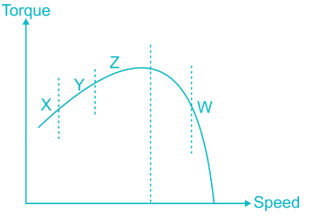Solution:

In regions X, Y and Z, as the speed increases the load torque on the induction motor is also increases and slip decreases. Hence all these three regions are unstable.

In region W, as the speed increases the load torque on the induction motor is decreases and slip increases. Hence this region is stable.

QUESTION: 14

What is the use of the circuit shown in the figure below?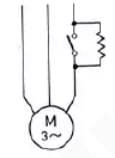Solution:

Resistance is connected in one phase of three phase induction motor is to provide smooth starting to the motor.

QUESTION: 15

As compared to a split phase motor, a capacitor start motor has _____.

Solution:

As compared to a split phase motor, a capacitor start motor has higher starting torque.

The order of starting torque in the increasing order is

Split phase motor < Capacitor start motor < Capacitor start capacitor run motor

QUESTION: 16

Why is the air gap between the yoke and armature of an electric motor kept smaller?

Solution:

The magnetic field always chooses the low reluctance path hence to achieve a strong magnetic field we need to maintain low reluctance path. It can be achieved by keeping smaller air gap between the yoke and armature of an electric motor.

QUESTION: 17

For a particular kW rating of an induction motor, the kVAR rating of the shunt capacitor required is _______.

Solution:

At higher rated speeds, slip is low. At lower slip values, power factor is high. Hence the required kVAR rating of the shunt capacitor is less for high rated speed motor.

At lower rated speeds, slip is high. At higher slip values, power factor is low. Hence the required kVAR rating of the shunt capacitor is more for lower rated speed motor.

QUESTION: 18

The induction motors develop which of the following at low frequency of the order ½ Hz to 10 Hz?

Solution:

The starting torque of an induction motor is given by,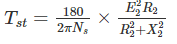The starting torque is inversely proportional to frequency.

At low frequency operation of induction motors, the starting torque will become high without any excessive starting current

QUESTION: 19

The open slots are more commonly used in the stator of a synchronous machine because _______.

A. The inductance of the winding is less

B. They facilitate the insertion of form wound and insulated coils

Solution:

Leakage reactance is less in open type slots. Therefore more amount of power will be transferred from stator to rotor and torque production is high. Windings can be placed in to the slots very easily.

QUESTION: 20

Three singly-excited electromagnetic structures are shown in the figures labelled I, II and III (R-Rotor, S-Stator), the reluctance torque can be developed in _______.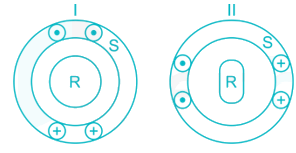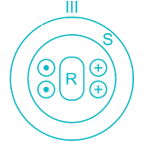Solution:

The reluctance torque produces when the airgap between the stator and rotor is not uniform.

Here in figure (I), the airgap is uniform, hence the reluctance torque cannot be developed.

In figure (II) and (III), airgap is not uniform, hence the reluctance torque can be developed.Use Code STAYHOME200 and get INR 200 additional OFF Use Coupon Code